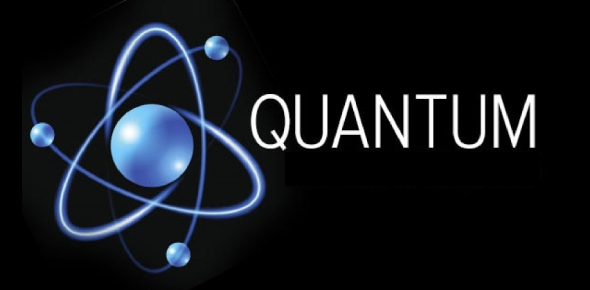# A Short Quantum Physics Quiz

Approved & Edited by ProProfs Editorial Team
At ProProfs Quizzes, our dedicated in-house team of experts takes pride in their work. With a sharp eye for detail, they meticulously review each quiz. This ensures that every quiz, taken by over 100 million users, meets our standards of accuracy, clarity, and engagement.
| Written by Rhartsock10
R
Rhartsock10
Community Contributor
Quizzes Created: 1 | Total Attempts: 15,511
Questions: 9 | Attempts: 15,526SettingsQuantum theory is the theoretical basis of modern physics that explains the nature and behavior of matter and energy on the atomic and subatomic levels. This quiz is designed to test the basic knowledge about quantum atomic theory and atomic orbitals. Give it a shot and be better prepared for your class tests!

• 1.

### The quantum mechanical model describes electrons as:

• A.

Particles

• B.

Waves

• C.

Particles with wave-like properties

• D.

Small, hard spheres

C. Particles with wave-like properties
Explanation
The Heisenberg uncertainty principle states that it is impossible to know both the position and momentum of an electron at the same time.

Rate this question:

• 2.

### Heisenberg’s Uncertainty Principle states that the ___ and ___ of an electron cannot be known simultaneously.

• A.

Position, mass

• B.

Position, charge

• C.

Momentum, speed

• D.

Position, momentum

D. Position, momentum
Explanation
The Heisenberg uncertainty principle states that it is impossible to know both the position and momentum of an electron at the same time.

Rate this question:

• 3.

### A wavefunction:

• A.

Is the solution to a differential equation known as a wave equation that describes the structure of an electron.

• B.

Is the solution to a differential equation known as a wave equation that describes the structure of an atom.

• C.

Is the differential equation used to describe the structure of an atom.

• D.

Is the differential equation used to describe the structure of an electron.

B. Is the solution to a differential equation known as a wave equation that describes the structure of an atom.
Explanation
A wave function is the solution to a differential equation known as a wave equation that describes the structure of an atom. Wave functions are another way of describing orbitals.

Rate this question:

• 4.

### There are ___ types of quantum numbers.

• A.

2

• B.

7

• C.

4

• D.

5

C. 4
Explanation
There are 4 types of quantum numbers: the principle quantum number, the angular momentum quantum number, the magnetic quantum number, and the spin quantum number. A complete set of quantum numbers (n, l, ml, and ms) completely describes an orbital.

Rate this question:

• 5.

### The principle quantum number is related to:

• A.

The shape of the orbital

• B.

The spatial orientation of the orbital

• C.

The average distance of the most electron-dense regions from the nucleus

• D.

The number of electrons

C. The average distance of the most electron-dense regions from the nucleus
Explanation
The principle quantum number is related to the average distance of the most electron-dense regions from the nucleus. Recall the figure in which, as n increases; the distance of the electron from the nucleus increases.

Rate this question:

• 6.

### The angular momentum quantum number is related to n by ___, and describes:

• A.

(n-1), the spatial orientation of the molecule

• B.

(n-1), the shape of the orbital

• C.

(n+1), the spatial orientation of the molecule

• D.

(n+1), the shape of the orbital

B. (n-1), the shape of the orbital
Explanation
The angular momentum quantum number is related to n by (n-1), and describes the shape of the orbital.

Rate this question:

• 7.

### The different types of subshells and corresponding number of orbitals is:

• A.

S:1, p:3, d:5, f:7

• B.

S:2, p:6, d:10, f:14

• C.

N:1, l:0, m:0

• D.

S:7, p:5, d:3, f:1

A. S:1, p:3, d:5, f:7
Explanation
The different types of subshells are s, p, d, and f, and correspond to 1, 3, 5, and 7 orbitals. Available orbitals could be 1 s-orbital, 3 p-orbitals, 5-d-orbitals, and 7 f-orbitals.

Rate this question:

• 8.

### The magnetic quantum number describes the:

• A.

Shape of the orbital.

• B.

Spatial orientation of the orbital.

• C.

Average distance of the most electron-dens regions from the nucleus.

• D.

Number of electrons.

B. Spatial orientation of the orbital.
Explanation
The magnetic quantum number describes the spatial orientation of the orbital. Recall that l defines the shape of the orbital, and ml defines the orientation with respect to a standard coordinate system.

Rate this question:

• 9.

### The spin quantum number (ms) can have a value of:

• A.

-1/2, +1/2

• B.

-1/2, -3/2

• C.

+1, -1

• D.

0, +1Back to top